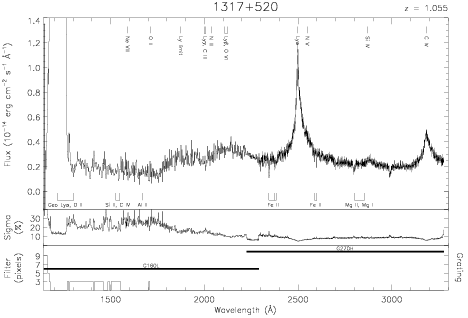Download dataset
TELESCOP= 'HST     '
INSTRUME= 'FOS     '
EQUINOX =               2000.0
DATE-OBS= '1993-07-08T01:35:16'
RA_PNT  =       199.9426250000
DEC_PNT =        51.8016944444
PA_PNT  =       234.7741320000
OBJECT  = '1317+520'
ROOTNM01= 'Y1HK0402T'
GRNDMD01= 'SPECTROSCOPY'
DETECT01= 'BLUE    '
APERID01= 'A-1     '
FGWAID01= 'L15     '
ROOTNM02= 'Y1HK0502T'
GRNDMD02= 'SPECTROSCOPY'
DETECT02= 'AMBER   '
APERID02= 'A-1     '
FGWAID02= 'H27     '